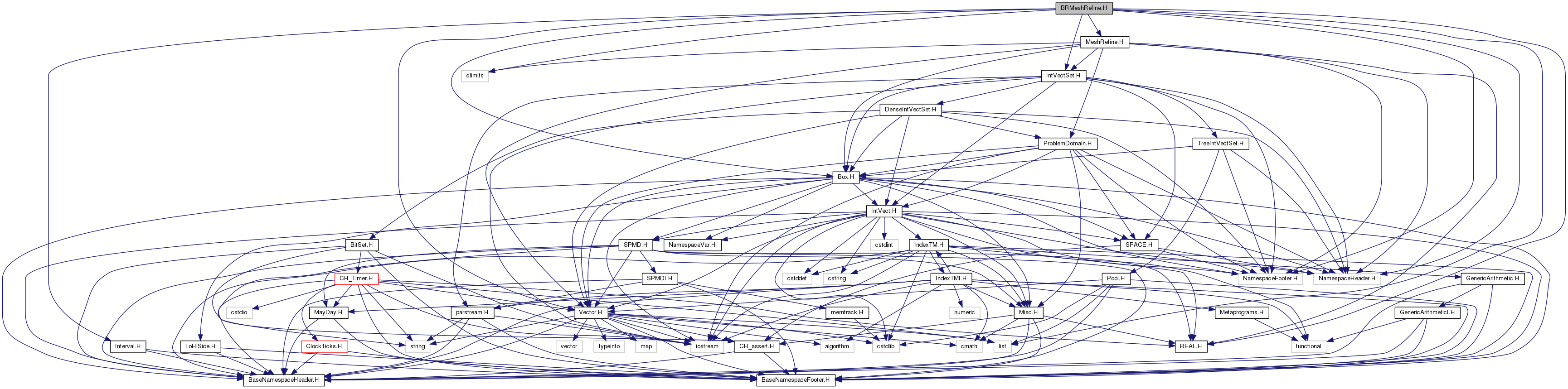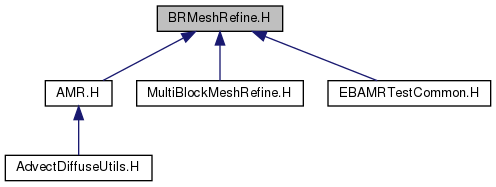Chombo + EB + MF  3.2
BRMeshRefine.H File Reference
`#include <climits>`
`#include "Vector.H"`
`#include "Box.H"`
`#include "IntVectSet.H"`
`#include "REAL.H"`
`#include "MeshRefine.H"`
`#include "Interval.H"`
`#include <list>`
`#include "NamespaceHeader.H"`
`#include "NamespaceFooter.H"`
Include dependency graph for BRMeshRefine.H:This graph shows which files directly or indirectly include this file:Go to the source code of this file.

## Classes

class  BRMeshRefine
Class which manages Berger-Rigoutsos grid generation. More...

## Macros

#define _BRMESHREFINE_H_

#define _BR_MIN_BOX_FILL_RATIO_   ( 0.75 )

## Functions

void domainSplit (const ProblemDomain &a_domain, Vector< Box > &a_vbox, int a_maxSize, int a_blockfactor=1, IntVect a_refineDirs=IntVect::Unit)
Splits domain into vector of disjoint boxes with max size maxsize. More...

void domainSplit (const Box &a_domain, Vector< Box > &a_vbox, int a_maxSize, int a_blockfactor=1, IntVect a_refineDirs=IntVect::Unit)

void breakBoxes (Vector< Box > &a_vboxin, const int &a_maxSize, const int &a_idir)

## Macro Definition Documentation

 #define _BRMESHREFINE_H_
 #define _BR_MIN_BOX_FILL_RATIO_   ( 0.75 )

## Function Documentation

 void domainSplit ( const ProblemDomain & a_domain, Vector< Box > & a_vbox, int a_maxSize, int a_blockfactor = `1`, IntVect a_refineDirs = `IntVect::Unit` )

Splits domain into vector of disjoint boxes with max size maxsize.

Blocking factor is default to one. If you make minimum size > 1, then domain must be coarsenable and refineable by blockfactor (refine(coarsen(domain,blockfactor), minsize) == domain) or an error is thrown. This would be defined in MeshRefine.H, except that it needs to use a BRMeshRefine object. Here a_refineDirs[d] is 1 if refining in dimension d, and 0 if not.

 void domainSplit ( const Box & a_domain, Vector< Box > & a_vbox, int a_maxSize, int a_blockfactor = `1`, IntVect a_refineDirs = `IntVect::Unit` )

Splits domain into a vector of disjoint boxes with maximum size maxsize. blocking factor is default to one. If you make minimum size > 1, then domain must be coarsenable and refineable by blockfactor (refine(coarsen(domain,blockfactor), minsize) == domain) or an error is thrown. This would be defined in MeshRefine.H, except that it needs to use a BRMeshRefine object. Here a_refineDirs[d] is 1 if refining in dimension d, and 0 if not.

 void breakBoxes ( Vector< Box > & a_vboxin, const int & a_maxSize, const int & a_idir )

Recursive function to enforce max size of boxes in a given direction. Does not call Meshrefine.